RS Aggarwal Class 9 Solutions Chapter 10 - Area Ex 10A (10.1)

Exercise 10.1: Area

Q.1: In the adjoining figure, ABCD is a quadrilateral. A line through D, parallel to AC, meets BC produced in P.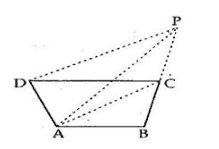Prove that: ar.( ABP$\triangle ABP$ ) = ar. (quad. ABCD)

Sol:Given: ABCD is a quadrilateral in which through D, a line is drawn parallel to AC which meets BC produced in P.

To prove: ar(ΔABP)=ar(quad.ABCD)$ar\left ( \Delta ABP \right )=ar\left ( quad.ABCD \right )$

Proof: ΔACPandΔACD$\Delta ACP \:and\:\Delta ACD$ have same base AC and lie between parallel lines AC and DP.

Therefore, ar(ΔACP)=ar(ΔACD)$ar\left ( \Delta ACP \right )=ar\left ( \Delta ACD \right )$

Adding ar(ΔACP)$ar\left ( \Delta ACP \right )$ on both sides, we get;

ar(ΔACP)+ar(ΔABC)=ar(ΔACD)+ar(ΔABC)$ar\left ( \Delta ACP \right )+ar\left ( \Delta ABC \right )= ar\left ( \Delta ACD \right )+ar\left ( \Delta ABC \right )$

Therefore, ar(ΔABP)=ar(quad.ABCD)$ar\left ( \Delta ABP \right )=ar\left ( quad.ABCD \right )$

Q.2: In the adjoining figure, AD and BC are 2 diagonals of the parallelogram ABCD. The point at which the diagonals intersect is O. Prove that OA = OD.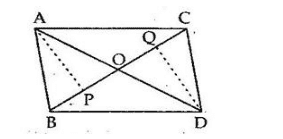Sol:

Given: Two triangles, i.e. ΔABCandΔDBC$\Delta ABC\:and\:\Delta DBC$ which have same base BC and points A and D lie on opposite sides of BC and ar(ΔABC)=ar(ΔDBC)$ar\left ( \Delta ABC \right )=ar\left ( \Delta DBC \right )$To prove:  OA = OD

Construction: Draw APBCandDQBC$AP\perp BC\:and\:DQ\perp BC$

Proof: We have:

ar(ΔABC)=12×BC×AP$ar\left ( \Delta ABC \right )=\frac{1}{2}\times BC\times AP$ and

ar(ΔBCD)=12×BC×DQ$ar\left ( \Delta BCD \right )=\frac{1}{2}\times BC\times DQ$

So, 1/2 x BC x AP = 1/2 x BC x DQ [From (1)]

Therefore, AP = DQ . . . . . . . . . (2)

Now, in ΔAOPandΔQOD$\Delta AOP\:and\:\Delta QOD$, we have:

APO=DQO=90$\angle APO=\angle DQO=90^{\circ}$

And, APO=DOQ=90$\angle APO=\angle DOQ=90^{\circ}$ [vertically opp. Angles]

AP = DQ [From (2)]

Thus, by Angle-Angle-side criterion of congruence, we have:

Therefore, ΔAOPΔQOD$\Delta AOP\cong \Delta QOD$ [AAS]

The corresponding parts of the congruent triangles are equal.

Therefore, OA = OD [C.P.C.T]

Q.3: In the adjoining figure, AD is one of the medians of a ABC$\triangle ABC$ and P is appointed on AD.

Prove that:

(i) ar.( BDP$\triangle BDP$) = ar.( CDP$\triangle CDP$)

(ii)ar.( ABP$\triangle ABP$ = ar.( ACP$\triangle ACP$)

Sol:

Given: A ΔABC$\Delta ABC$ in which AD is the median and P is a point on AD.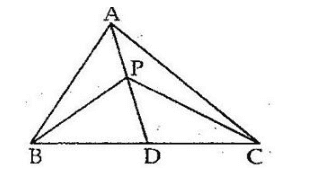To prove:

(i) ar(ΔBDP)=ar(ΔCDP)$ar\left ( \Delta BDP \right )=ar\left ( \Delta CDP \right )$

(ii) ar(ΔABP)=ar(ΔAPC)$ar\left ( \Delta ABP \right )=ar\left ( \Delta APC \right )$

Proof:

(i) In ΔBPC$\Delta BPC$, PD is the median. Since median of a triangle divides the triangle into two triangles of equal areas.

So, ar(ΔBDP)=ar(ΔCDP)$ar\left ( \Delta BDP \right )=ar\left ( \Delta CDP \right )$ . . . . . . . . (1)

(ii) In ΔABC$\Delta ABC$, AD is the median

So, ar(ΔABD)=ar(ΔADC)$ar\left ( \Delta ABD \right )=ar\left ( \Delta ADC \right )$

But, ar(ΔBDP)=ar(ΔCDP)$ar\left ( \Delta BDP \right )=ar\left ( \Delta CDP \right )$ [From (1)]

Subtracting ar(ΔBPD)$ar\left ( \Delta BPD \right )$ from both the sides of the equation, we have

Therefore, ar(ΔABD)ar(ΔBPD)=ar(ΔADC)ar(ΔBPD)$ar\left ( \Delta ABD \right )-ar\left ( \Delta BPD \right )=ar\left ( \Delta ADC \right )-ar\left ( \Delta BPD \right )$

=ar(ΔBPD)ar(ΔCDP)$=ar\left ( \Delta BPD \right )-ar\left ( \Delta CDP \right )$ [From (1)]

Therefore, ar(ΔABP)=ar(ΔACP)$ar\left ( \Delta ABP \right )=ar\left ( \Delta ACP \right )$

Q.4: In the adjoining figure, the diagonals AC and BD of a quadrilateral ABCD intersect at O.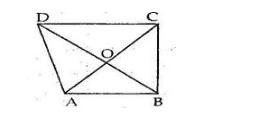If BO = OD, prove that

ar.( ABC$\triangle ABC$)=ar. (ADC$\triangle ADC$).

Sol:

Given: A quadrilateral ABCD in which diagonals AC and BD intersect at O and BO = ODTo Prove: ar(ΔABC)=ar(ΔADC)$ar\left ( \Delta ABC \right )=ar\left ( \Delta ADC \right )$

Proof: Since OB = OD [given]

So, AO is the median of ΔABD$\Delta ABD$

Therefore, ar(ΔAOD)=ar(ΔAOB)$ar\left ( \Delta AOD \right )=ar\left ( \Delta AOB \right )$ . . . . . . . . (i)

As OC is the median of ΔCBD$\Delta CBD$

Therefore, ar(ΔDOC)=ar(ΔBOC)$ar\left ( \Delta DOC \right )=ar\left ( \Delta BOC \right )$ . . . . . . . . . (ii)

Adding both sides of (i) and (ii), we get:

ar(ΔAOD)+ar(ΔDOC)=ar(ΔAOB)+ar(ΔBOC)$ar\left ( \Delta AOD \right )+ar\left ( \Delta DOC \right )= ar\left ( \Delta AOB \right )+ar\left ( \Delta BOC \right )$

Therefore, ar(ΔADC)=ar(ΔABC)$ar\left ( \Delta ADC \right )=ar\left ( \Delta ABC \right )$

Q.5: ABC is a triangle in which D is the midpoint of BC and E is the midpoint of AD.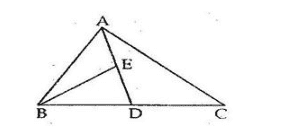Prove that:

ar.( BED$\triangle BED$)= 14ar(ABC)$\frac{1}{4}ar(\triangle ABC)$

Sol:

Given: A ΔABC$\Delta ABC$ in which AD is a median and E is the mid-point of ADTo prove: ar(ΔBED)=14ar(ΔABC)$ar\left ( \Delta BED \right )=\frac{1}{4}ar\left ( \Delta ABC \right )$

Proof: Since, ar(ΔABD)=ar(ΔACD)$ar\left ( \Delta ABD \right )=ar\left ( \Delta ACD \right )$   [Since,ADisamedian]$\left [Since, AD\:is\:a\:median \right ]$

i.e. ar(ΔABD)=12ar(ΔABC)$ar\left ( \Delta ABD \right )=\frac{1}{2}ar\left ( \Delta ABC \right )$ . . . . . . . (1) [Since,ar(ΔABC)=ar(ΔABD)+ar(ΔADC)]$\left [ Since, ar\left ( \Delta ABC \right )=ar\left ( \Delta ABD \right ) +ar\left ( \Delta ADC \right )\right ]$

Now, as BE is the median of ΔABD$\Delta ABD$

ar(ΔABE)=ar(ΔBED)$ar\left ( \Delta ABE \right )=ar\left ( \Delta BED \right )$ . . . . . . . . . (2)

Since, ar(ΔABD)=ar(ΔABE)+ar(ΔBED)$ar\left ( \Delta ABD \right )=ar\left ( \Delta ABE \right ) +ar\left ( \Delta BED \right )$ . . . . . . . (3)

Therefore, ar(ΔBED)=ar(ΔABE)$ar\left ( \Delta BED \right )=ar\left ( \Delta ABE \right )$……..[From(2)]

= 12ar(ΔABD)$\frac{1}{2}ar\left ( \Delta ABD \right )$  [From (2) and (3)]

= 12[12ar(ΔABC)]$\frac{1}{2}\left [ \frac{1}{2}ar\left ( \Delta ABC \right ) \right ]$  [From (1)]

= 14ar(ΔABC)$\frac{1}{4}ar\left ( \Delta ABC \right )$

Q.6: The vertex A of ABC$\triangle ABC$ is joined to a point D on the side BC. The midpoint AD is E.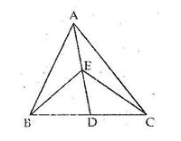Prove that:

ar(BEC$\triangle BEC$) = 12ar(ABC)$\frac{1}{2}ar(\triangle ABC)$

Sol:

Given: A ΔABC$\Delta ABC$ in which E is the mid-point of the line segment AD where D is a point on BC.

To Prove: ar(ΔBEC)=12ar(ΔABC)$ar\left ( \Delta BEC \right )=\frac{1}{2}ar\left ( \Delta ABC \right )$

Proof: Since, BE is a median of ΔABD$\Delta ABD$

So, ar(ΔBDE)=ar(ΔABE)$ar\left ( \Delta BDE \right )=ar\left ( \Delta ABE \right )$

i.e. ar(ΔBDE)=12ar(ΔABD)$ar\left ( \Delta BDE \right )=\frac{1}{2}ar\left ( \Delta ABD \right )$ . . . . . . . (1)

As, CE is the median of ΔADC$\Delta ADC$

So, ar(ΔCDE)=12ar(ΔACD)$ar\left ( \Delta CDE \right )=\frac{1}{2}ar\left ( \Delta ACD \right )$ . . . . . . . (2)

Adding (i) and (ii), we get:

ar(ΔBDE)+ar(ΔCDE)=12ar(ΔABD)+12ar(ΔACD)$ar\left ( \Delta BDE \right )+ar\left ( \Delta CDE \right )=\frac{1}{2}ar\left ( \Delta ABD \right )+\frac{1}{2}ar\left ( \Delta ACD \right )$
ar(ΔBDE)=12[ar(ΔABD)+ar(ΔACD)]$ar\left ( \Delta BDE \right )=\frac{1}{2}\left [ ar\left ( \Delta ABD \right )+ar\left ( \Delta ACD \right ) \right ]$

= 12ar(ΔABC)$\frac{1}{2}ar\left ( \Delta ABC \right )$

Q.7: D is the midpoint of side BC of ABC$\triangle ABC$ and E is the midpoint of BD. if O is the midpoint of AE.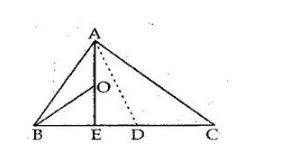Prove that:

ar.( BOE$\triangle BOE$ ) = 18ar(ABC)$\frac{1}{8}ar(\triangle ABC)$

Sol:

Given: A ΔABC$\Delta ABC$ in which AD is the medium and E is the mid-point of BD. O is the mid-point of AE.

To prove: ar(ΔBOE)=18ar(ΔABC)$ar\left ( \Delta BOE \right )=\frac{1}{8}ar\left ( \Delta ABC \right )$

Proof: Since O is the mid-point of AE.

So, BO is the medium of ΔBAE$\Delta BAE$

Therefore, ar(ΔBOE)=12ar(ΔABE)$ar\left ( \Delta BOE \right )=\frac{1}{2}ar\left ( \Delta ABE \right )$ . . . . (1)

Now, E is the mid-point of BD

So AE divides ΔABD$\Delta ABD$ into two triangles of equal area.

Therefore, ar(ΔABE)=12ar(ΔABD)$ar\left ( \Delta ABE \right )=\frac{1}{2}ar\left ( \Delta ABD \right )$ . . . .(2)

As D is the mid-point of BC

So, ar(ΔABD)=12ar(ΔABC)$ar\left ( \Delta ABD \right )=\frac{1}{2}ar\left ( \Delta ABC \right )$ . . . . . (3)

Therefore, ar(ΔBOE)=12ar(ΔABE)$ar\left ( \Delta BOE \right )=\frac{1}{2}ar\left ( \Delta ABE \right )$ [From (1)]

= 12[12ar(ΔABCD)]$\frac{1}{2}\left [ \frac{1}{2}ar\left ( \Delta ABCD\right ) \right ]$ [From (2)]

= 14ar(ΔABD)$\frac{1}{4}ar\left ( \Delta ABD \right )$

= 14×12ar(ΔABC)$\frac{1}{4}\times \frac{1}{2}ar\left ( \Delta ABC \right )$ [From (3)]

= 18ar(ΔABC)$\frac{1}{8}ar\left ( \Delta ABC \right )$

Q.8: In the adjoining figure, ABCD parallelogram and O is any point on the diagonal AC.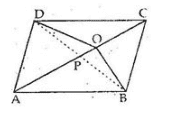Show that: ar.( AOB$\triangle AOB$ )=ar.( AOD$\triangle AOD$)

Sol:

Given: A parallelogram ABCD in which O is any point on the diagonal ACTo Prove: ar(ΔAOB)=ar(ΔAOD)$ar\left ( \Delta AOB \right )=ar\left ( \Delta AOD \right )$.

Construction: Join BD which intersects AC at P.

Proof: As diagonals of a parallelogram bisect each other therefore, OP is the median of ΔODB$\Delta ODB$

Therefore, ar(ΔODP)=ar(ΔOBP)$ar\left ( \Delta ODP \right )=ar\left ( \Delta OBP \right )$

Also, AP is the median of ΔABD$\Delta ABD$

Therefore, ar(ΔODP)=ar(ΔOBP)$ar\left ( \Delta ODP \right )=ar\left ( \Delta OBP \right )$

ar(ΔODP)+ar(ΔADP)=ar(ΔOBP)+ar(ΔABP)$ar\left ( \Delta ODP \right )+ar\left ( \Delta ADP \right )=ar\left ( \Delta OBP \right )+ar\left ( \Delta ABP \right )$

Therefore, ar(ΔAOB)=ar(ΔAOD)$ar\left ( \Delta AOB \right )=ar\left ( \Delta AOD \right )$.

Q.9: P, Q, R, S are respectively the midpoints of the sides AB, BC, CD and DA of parallelogram ABCD. Show that PQRS is parallelogram and also show that

ar.(parallelogram PQRS)= 12$\frac{1}{2}$ * ar.(parallelogram ABCD).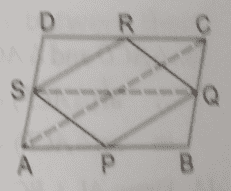Sol:

Given: ABCD is a parallelogram and P,Q,R and S are the midpoints of AB,BC,CD,DA respectively.

To prove: PQRS is a parallelogram and parallelogram PQRS= 12$\frac{1}{2}$ Parallelogram ABCD

Construction: Join AC,BD and SQ.

Proof: As S and R are the midpoints of AD and CD, so in ADC$\bigtriangleup ADC$

SRAC$SR\parallel AC$ ( By midpoint theorem)

Also, as P and Q are the midpoints of AB and BC. So, in ABC$\bigtriangleup ABC$,

PQAC$PQ \parallel AC$

Therefore, PQACSR$PQ \parallel AC \parallel SR$

So, PQSR$PQ \parallel SR$

Similarly, we can prove SPRQ$SP \parallel RQ$.

Thus PQRS is a parallelogram as its opposite sides are parallel.

Since the diagonals of a parallelogram bisect each other.

So in ABD$\bigtriangleup ABD$,

O is the midpoint of AC and S is the midpoint of AD.

Therefore, OSAB$OS\parallel AB$ (by midpoint theorem )

Similarly in ABC$\bigtriangleup ABC$ we can prove that,

OQAB$OQ\parallel AB$ and SQAB$SQ\parallel AB$

Thus, ABQS is a parallelogram.

Now, SPQ=12(parallelogramABQS)$\bigtriangleup SPQ =\frac{1}{2}(parallelogram \; ABQS)$ . . . . . . . (i)

Similarly, we can prove that:

SPQ=12(parallelogramSQCD)$\bigtriangleup SPQ =\frac{1}{2}(parallelogram \; SQCD)$ . . . . . . . (ii)

Adding (i) and (ii) we get,

SPQ+SPQ=12(parallelogramABQS)+12(parallelogramSQCD)$\bigtriangleup SPQ + \bigtriangleup SPQ =\frac{1}{2}(parallelogram \; ABQS) + \frac{1}{2}(parallelogram \; SQCD)$

Therefore, (parallelogramPQRS)=12(parallelogramABCD)$(parallelogram \; PQRS)= \frac{1}{2}(parallelogram \; ABCD)$

Q.10: The given figure shows a pentagon ABCDE. EG, drawn parallel to DA, meets BA produced at G, and CF, drawn parallel to DB, meets AB produced at F. Show that ar.(pentagon ABCDE) = ar.( DGF$\triangle DGF$).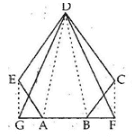Sol:

Given: ABCDE is a pentagon, EDDA$ED\parallel DA$ and CFDB$CF\parallel DB$.

To prove: ar(pentagon ABCDE) = ar(DGF$\bigtriangleup DGF$)Proof:

ar(pentagon ABCDE) = ar(DBC$\bigtriangleup DBC$) +ar(ADE$\bigtriangleup ADE$)+ar(ABD$\bigtriangleup ABD$) . . . . . . . . (i)

Also, ar(DGF$\bigtriangleup DGF$)= ar(DBF$\bigtriangleup DBF$)+ar(ADG$\bigtriangleup ADG$)+ ABD$\bigtriangleup ABD$ . . . . . (ii)

Now, DBC$\bigtriangleup DBC$ and DBF$\bigtriangleup DBF$ lie on the same base and between the same parallel lines.

Therefore, ar(DBC)=ar(DBF)$ar(\bigtriangleup DBC)=ar(\bigtriangleup DBF)$ . . . . . . (iii)

Similarly, ADE$\bigtriangleup ADE$ and ADG$\bigtriangleup ADG$ lie on the same base and between the same parallel lines.

Therefore, ar(ADE)=ar(ADG)$ar(\bigtriangleup ADE)=ar(\bigtriangleup ADG)$ . . . . . . . (iv)

From (iii) and (iv) we have:

ar(DBC)+ar(ADE)=ar(DBF)+ar(ADG)$ar(\bigtriangleup DBC)+ ar(\bigtriangleup ADE) =ar(\bigtriangleup DBF) +ar(\bigtriangleup ADG)$

Adding ar(ABD)$ar(\bigtriangleup ABD)$ on both sides

ar(DBC)+ar(ADE)+ar(ABD)=ar(DBF)+ar(ADG)+ar(ABD)$ar(\bigtriangleup DBC)+ ar(\bigtriangleup ADE) + ar(\bigtriangleup ABD) =ar(\bigtriangleup DBF) +ar(\bigtriangleup ADG) + ar(\bigtriangleup ABD)$

By substituting the values from (i) and (ii) we get:

ar(pentagon ABCDE) = ar(DGF$\bigtriangleup DGF$)

Q.11: Prove that a median divides a triangle into two triangles of equal area.

Sol:

Let, AD is a median of ABC$\bigtriangleup ABC$ and D is the midpoint of BC. AD divides ABC$\bigtriangleup ABC$ in two triangles :ABDandADC$\bigtriangleup ABD \; and \; \bigtriangleup ADC$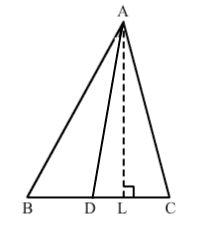To prove: ar(ABD)=ar(ADC)$ar(\bigtriangleup ABD)=ar(\bigtriangleup ADC)$

Construction: Draw ALBC$AL\perp BC$

Proof:

Since, D is the midpoint of BC, we have

BD=DC$BD=DC$

Multiplying with 12AL$\frac{1}{2}AL$ on both sides, we have

12×BD×AL=12×DC×AL$\frac{1}{2} \times BD\times AL=\frac{1}{2}\times DC\times AL$

Therefore, ar(ABD)=ar(ADC)$ar(\bigtriangleup ABD)=ar(\bigtriangleup ADC)$

Q.12: Show that a diagonal divides a parallelogram into two triangles of equal area.

Sol: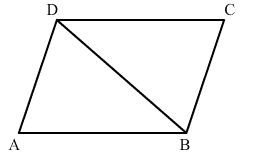Let, ABCD be a parallelogram and BD be its diagonal.

To prove: ar(ABD)=ar(CDB)$ar(\bigtriangleup ABD)=ar(\bigtriangleup CDB)$

Proof:

In ABDandCDB$\bigtriangleup ABD \; and \; \bigtriangleup CDB$ we have,

AB = CD (opposite sides of a parallelogram)

AD = CD (opposite sides of a parallelogram)

BD = DB (common side)

Therefore, ABDCDB$\bigtriangleup ABD\cong \bigtriangleup CDB$ (SSS criteria )

ar(ABD)=ar(CDB)$ar(\bigtriangleup ABD)=ar(\bigtriangleup CDB)$

Q.13: The base BC of ABC$\triangle ABC$ is divided at D such that BD = 12DC$\frac{1}{2}DC$ . Prove that

ar( ABD$\triangle ABD$) = 13×ar(ABC)$\frac{1}{3}\times ar(\triangle ABC)$

Sol: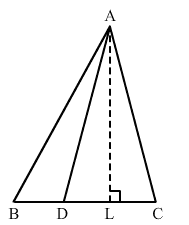Given: D is a point on BC of ABC$\bigtriangleup ABC$, such that BD=12DC$BD=\frac{1}{2}DC$

To prove: ar(ABD)=13ar(ABC)$ar(\bigtriangleup ABD)=\frac{1}{3}ar(\bigtriangleup ABC)$

Construction: Draw ALBC$AL\perp BC$

Proof:

In ABC$\bigtriangleup ABC$, we have :

BC = BD + DC

BD+2BD=3×BD$\Rightarrow BD+2 BD =3\times BD$

Now we have:

ar(ABD)=12×BD×AL$ar(\bigtriangleup ABD)=\frac{1}{2}\times BD\times AL$
ar(ABC)=12×BC×AL$ar(\bigtriangleup ABC)=\frac{1}{2}\times BC\times AL$
ar(ABC)=12×3BD×AL=3(12×BD×AL)$\Rightarrow ar(\bigtriangleup ABC)=\frac{1}{2}\times 3BD\times AL=3\left (\frac{1}{2}\times BD\times AL \right )$
ar(ABC)=3×ar(ABD)$\Rightarrow ar(\bigtriangleup ABC)=3\times ar(\bigtriangleup ABD)$

Therefore, ar(ABD)=13ar(ABC)$ar(\bigtriangleup ABD) = \frac{1}{3} ar (\bigtriangleup ABC)$

Q.14: In the adjoining figure, the point D divides the side BC of ABC$\triangle ABC$ in the ratio m : n.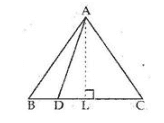Prove that:

ar(ABD$\triangle ABD$):ar(ADC$\triangle ADC$) = m:n.

Sol:Given: D is a point on BC of ABC$\bigtriangleup ABC$ such that BD:DC=m:n$BD:DC= m: n$

To prove: ar(ABD):ar(ADC)=m:n$ar(\bigtriangleup ABD):ar (\bigtriangleup ADC)= m:n$

Construction : Draw ALBC$AL\perp BC$

Proof:

ar(ABD)=12×BD×AL$ar(\bigtriangleup ABD )=\frac{1}{2}\times BD\times AL$ . . . . . . (i)

ar(ABD)=12×DC×AL$ar(\bigtriangleup ABD )=\frac{1}{2}\times DC \times AL$ . . . . . . (ii)

Dividing (i) and (ii) we have:

ar(ABD)ar(ABC)=12×BD×AL12×DC×AL$\frac{ar(\bigtriangleup ABD )}{ar(\bigtriangleup ABC )}=\frac{\frac{1}{2}\times BD\times AL}{\frac{1}{2}\times DC \times AL}$

=BDDC$=\frac{BD}{DC}$ = mn$\frac{m}{n}$

Therefore, ar(ABD):ar(ADC)=m:n$ar(\bigtriangleup ABD):ar (\bigtriangleup ADC)= m:n$

Practise This Question

Energy is the ability of doing work.